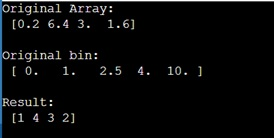# numpy.digitize() Method with Example

Python | numpy.digitize(): Learn about the numpy.digitize() method, its usages and example.
Submitted by Pranit Sharma, on February 27, 2023

NumPy is an abbreviated form of Numerical Python. It is used for different types of scientific operations in python. Numpy is a vast library in python which is used for almost every kind of scientific or mathematical operation. It is itself an array which is a collection of various methods and functions for processing the arrays.

## numpy.digitize() Method

The numpy.digitize() is used to return the indices of the bins to which each value in the input array belongs.

The concept of bins in digitize() method is like a range between which a value of numpy array may lie. There is a parameter in digitize method called right which means in a certain range, we want the higher value to be considered or not.

Suppose that we are having a bin array as [ 1,2,3,4 ] and an input array as [ 1.5], now since 1.5 lies between 1 and 2 and if we do not consider the higher values of the range 1 and 2, it will return the index of 1 that is 0.

In a similar fashion, numpy.digitize() method works. This type of discretization allows us to apply algorithms designed for discrete spaces such as Sarsa, Sarsamax, or Expected Sarsa to continuous spaces.

Syntax:

```numpy.digitize(x, bins, right=False)
```

Parameter(s):

• x: an input array
• bins: an array of ranges
• right: Indication about the consideration of the right value or not.

Let us understand with the help of an example,

## Python code to demonstrate the example of numpy.digitize() method

```# Import numpy
import numpy as np

# Creating a numpy array
arr = np.array(np.array([0.2, 6.4, 3.0, 1.6]))

# Display original array
print("Original Array:\n",arr,"\n")

# Creating a bin
bin = np.array([0.0, 1.0, 2.5, 4.0, 10.0])

# Display original bin
print("Original bin:\n",bin,"\n")

# Using numpy.digitize
res = np.digitize(arr,bin)

# Display result
print("Result:\n",res)
```

Output:Languages: » C » C++ » C++ STL » Java » Data Structure » C#.Net » Android » Kotlin » SQL
Web Technologies: » PHP » Python » JavaScript » CSS » Ajax » Node.js » Web programming/HTML
Solved programs: » C » C++ » DS » Java » C#
Aptitude que. & ans.: » C » C++ » Java » DBMS
Interview que. & ans.: » C » Embedded C » Java » SEO » HR
CS Subjects: » CS Basics » O.S. » Networks » DBMS » Embedded Systems » Cloud Computing
» Machine learning » CS Organizations » Linux » DOS
More: » Articles » Puzzles » News/Updates